# NCERT Solutions for Class 4 Maths Fields And Fences

NCERT solutions for Class 4 Mathematics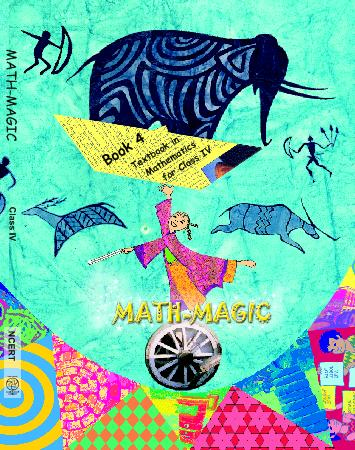## NCERT Solutions for Class 4 Maths Fields And Fences

Chapter -13 Class 4 Maths Fields And Fences

### 1. I need a fence around my field. How much wire should I buy.

Ans. The length of the wire required for fencing the field is equal to the boundary of the field.

### 2. Can you find it from this picture?

Ans. Yes, I can find its boundary. It is equal to 21m + 15m + 9m + 9m = 54m of the field.

### 3. How much wire did Rahmat give Ganpat?

Ans. Rahmat gave Ganpat a wire of length 70m – 54 m = 16m.

### 4. How long is the boundary of Ganpat’s field?

Ans. Boundary of the Ganpat’s field = 18m + 9m + 15m + 15m + 9m = 66 metres.

### 5. How much wire will Ganpat need for his field?

Ans. Ganpat’s total requirement of wire = 66 metres

Length of wire given by Rahmat = 16 metres

Therefore, the length of more wire needed by Ganpat = (66-16) = 50 metres.

### 6. Here are the picture of some more fields. Find out which one has the biggest boundary.

(a)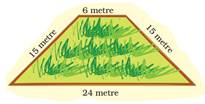Ans. Boundary = (24 + 15 + 6 + 15) metres= 60 metres

(b)Ans. Boundary = (12 + 6 +6 + 3 +6 + 9) metres = 42 metres.

(c)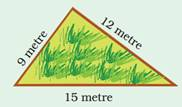Ans. Boundary = (15 + 12 + 9) metres = 36 metres.

(d)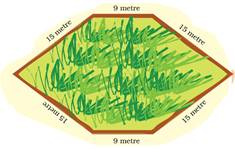Ans. (d) Boundary = (9 + 15 + 15 + 9 + 15 + 15) metres = 78 metres.

### 7. Chandu’s father is called the “young old man” in his village. At 70 years age, he is fully fit. Do you know his secret? He goes for a walk around the field every morning. Everyday he takes four rounds of Chandu’s fields. What is the total distance he covers.

4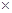___ = _____ m = ________ km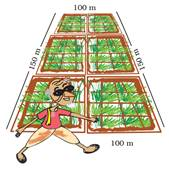Ans. Boundary of Chandu’s field = 100 m + 150 m + 100 m + 150 m = 500m.

Total Distance covered by Chandu’s father = 4Boundary of Chandu’s field

= 4500m = 2000 m = 2 km.

### 8. Look at the picture of the table cloth and tell how much is used for one table cloth.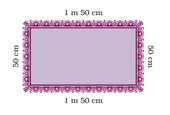Ans. Length of lace = 2(1m 50 cm + 50 cm) = 22m = 4m.

### 9. How much lace will be used in 3 such table clothes? How much lace will be left in the roll?

Ans. Lace used for one table cloth = 1m 50 cm + 50 cm + 1m 50 cm + 50 cm = 4metres

Lace used for 3 such table cloths = 34 Lace used for on one table cloth

= 34 metres = 12 metres.

Lace left in the roll = Total Lace – Lace used = 100m – 12m = 88m.

### 10. Find out the length of the boundary of these shapes.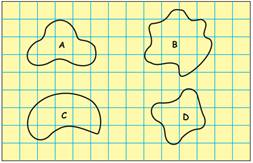Ans. To find the length of the boundary, take a long piece of thread and carefully place it along the boundary of the shape. Cut out the exact length of the thread needed for covering the shape, starting from one point and coming back to the same point. On measuring the length of this thread, we obtain the length of this thread, we obtain boundary of the shape. Repeat the process for each shape.

### 11. Now count the square and find out:

(a) How many squares are there in each shape?

Ans. The number of complete squares in shape A is 1, in shape B is 2, in shape C is 3 and shape D is 2.

(b) Which shape covers the least number of squares?

Ans. The least number of complete squares are in shape A.

(c) Which shape covers the most number of squares?

Ans. Shape C has the most number of complete squares.

### 12. A square has a boundary of 12 cm.

(a) From the corner of this square, a small square of side 1 cm is cut off. Will the boundary of B be less or more? Find its length.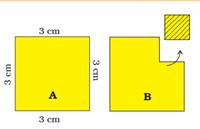Ans. Boundary of B = 3cm + 2 cm + 1 cm + 2 cm +3 cm = 12cm

Since the boundary of A is also 12 cm.

So, the boundary of B is neither less nor more than that of A. But their boundaries are equal.

(b) If you cut 1 cm square to get shape C what will be the length of the boundary of C?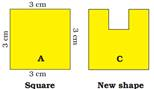Ans. Boundary of C = 3cm + 3cm + 1cm + 1cm + 1 cm +1cm + 1cm + 3 cm = 14 cm.

### 13. (a) Find the length of the boundary of square D.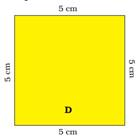Ans. Length of the boundary of square D = 5cm + 5cm + 5cm + 5cm = 20cm

(b) The boundary of this square (1cm) is ___ + ____ + ____ + ____.

Ans. The boundary of this square (1cm) is 1cm + 1cm+ 1cm + 1cm = 4cm.

Yes, we can also say that the boundary is 41 cm.

### 14. A hockey field is 91 metres 40cm and 55 metres wide. How long is the boundary of the field?

Ans. Length of the boundary of a hockey field = 91m + 40cm +55m + 91m 40 cm +55m

= 292 m 80 cm

Class 4 Maths Fields And Fences

### 15. Usha and Valsamma are running a race. Usha is running on the inner circle. Valsamma is running on the outer circle. Valsamma runs after than Usha. But still loses the race. Can you guess why?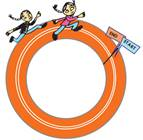Ans. Since inner boundary is smaller than the outer boundary and as such Valsamma has to run for more distance, therefore, she loses the race.

### 16.Have you seen any race where runners start from different places – like in this picture? Guess why?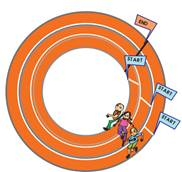Ans. In order to make their running distances equal.

### 17. How will Neetu find out if the two gardens are equally big?

Ans. To find out if the two gardens are equally big:

Let us cover each garden with cardboards of same size without overlapping. We see that the same number of cardboards cover each garden. Therefore, we can say that the two gardens are equally big.

### 18. (a) How many small squares of size 1cm are there in this big green square?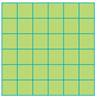Ans. There are 36 small squares of size 1 cm in the big green square.

(b) Can you think of a faster way to know the total number of small square without counting it?

Ans. Yes, there is a faster way to find the total number of small square. Just find: 66=36.

Class 4 Maths Fields And Fences

### 19. Guess how many squares of one centimetre can fill this rectangle? Checking your guess by completing the grid.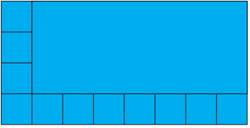Ans. By completing the grid with squares of one centimetre, we find the number of such squares is 32.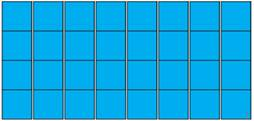## NCERT solutions for Class 4 Mathematics Chapter 13 Fields And Fences

Building with BricksView Solutions
Long and ShortView Solutions
A trip to BhopalView Solutions
Tick Tick TickView Solutions
The Way the World LooksView Solutions
The Junk SellerView Solutions
Jugs and MugsView Solutions
Carts and WheelsView Solutions
Halves and QuartersView Solutions
Play With PatternsView Solutions
Table and SharesView Solutions
How Heavy How LightView Solutions
Fields And FencesView Solutions
Smart ChartsView Solutions

NCERT Solutions Class 4 Maths Fields And Fences PDF (Download) Free from myCBSEguide app and myCBSEguide website. Ncert solution class 4 Maths includes text book solutions from Class 4 Maths Book . NCERT Solutions for CBSE Class  Maths have total 14 chapters. 4 Maths NCERT Solutions in PDF for free Download on our website. Ncert Maths class 4 solutions PDF and Maths ncert class 4 PDF solutions with latest modifications and as per the latest CBSE syllabus are only available in myCBSEguide.

## CBSE app for Students

To download NCERT Solutions for Class 4 Maths, EVS Hindi English, Maths Science do check myCBSEguide app or website. myCBSEguide provides sample papers with solution, test papers for chapter-wise practice, NCERT solutions, NCERT Exemplar solutions, quick revision notes for ready reference, CBSE guess papers and CBSE important question papers. Sample Paper all are made available through the best app for CBSE students and myCBSEguide website.### Test Generator

Create question paper PDF and online tests with your own name & logo in minutes.### myCBSEguide

Question Bank, Mock Tests, Exam Papers, NCERT Solutions, Sample Papers, Notes

### 4 thoughts on “NCERT Solutions for Class 4 Maths Fields And Fences”

1. Very nice thx for this,

2. Very helpful thank you so much

3. It is very helpful for my kid